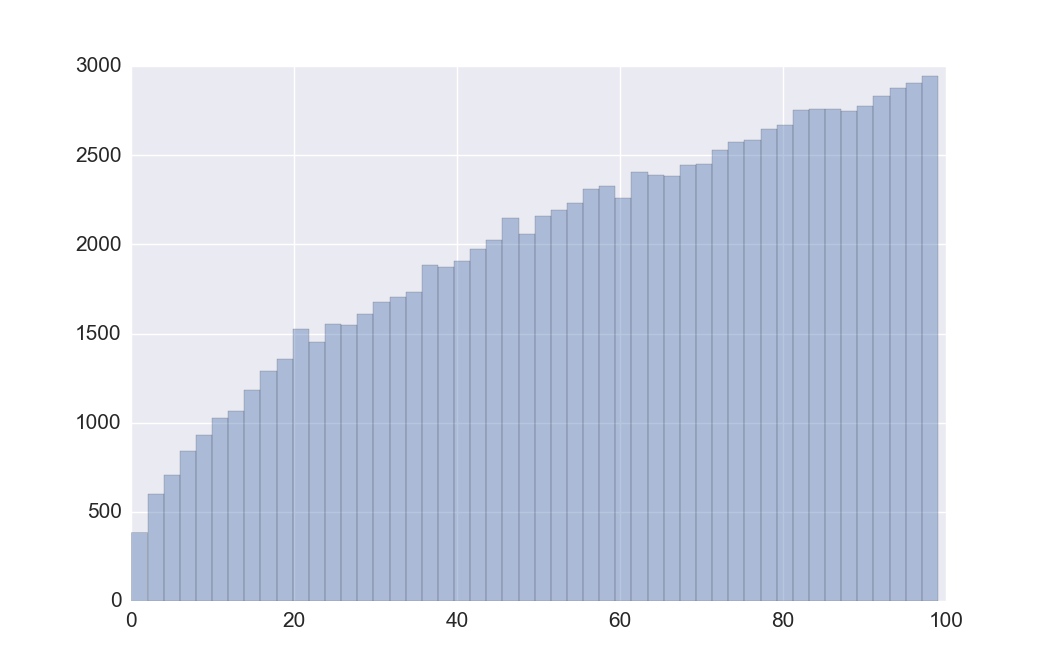# 蓄水池抽样浅说 (3)

#### 加个权吧

$$P_i = \frac{w_i}{w_1 + w_2 + \cdots + w_n}$$

$$P_n(1) = \frac{w_n}{w_1 + w_2 + \cdots + w_n}$$

$$P_{n-1}(2) = \frac{w_{n-1}}{w_1 + w_2 + \cdots + w_{n-1}}$$

$$P(S) = \prod_{i=1}^n \frac{w_i}{w_1+w_2+\cdots w_i}$$

def reservoirSampleAES(stream, sample_size):
result = []
for index, line in enumerate(stream):
w = (index + 1)** (1/2)
key = random.random() ** (1/w)
if index < sample_size:
heapq.heappush(result, (key, line))
elif key > heapq.nsmallest(1, result):
heapq.heappushpop(result, (key, line))
return list(zip(*result))

import matplotlib.pyplot as plt
a = []
for i in range(10000):
a.extend(reservoirSample(range(100), 10))
plt.hist(a, 100)
plt.show()$$\mathbb{P}[X_1 \leq X_2 \leq \cdots \leq X_n \leq 1] = \prod_{i=1}^n \frac{w_i}{w_1+w_2+\cdots w_i}$$

$$F_{X_i}(t) = \mathbb{P}[X_i \leq t] = \mathbb{P}\big[ U_i^{1/w_i}\leq t\big] = \mathbb{P}\big[U_i \leq t^{w_i}\big] = t^{w_i}$$

$$f_{X_i}(t) = F_{X_i}'(t) = w_i\cdot t^{w_i -1}$$

$n=1$ 时，$\mathbb{P}[U_1 \leq \alpha^{w_1}] = \alpha^{w_1}$

$n=2$ 时，

\begin{align} \mathbb{P}[U_1 \leq U_2 \leq \alpha^{w_1}] &= \int_0^\alpha F_{X_1}(t)\,f_{X_2}(t) \mathrm{d}t\\ &=\int_0^\alpha t^{w_1}\cdot w_2 t^{w_2-1}\mathrm{d}t = \frac{w_2}{w_1 + w_2}\cdot \alpha^{w_1+w_2} \end{align}

\begin{align} &\phantom{==} \mathbb{P}[X_1 \leq X_2 \leq \cdots \leq X_{k+1} \leq \alpha] \\ &= \int_0^\alpha \mathbb{P}[X_1 \leq X_2 \leq \cdots \leq X_k \leq t]\cdot f_{X_{k+1}}(t) \mathrm{d}t\\ &=\int_0^\alpha \left(\prod_{i=1}^k \frac{w_i}{w_1+w_2+\cdots w_i}\right) t^{\sum_{i=1}^k w_i}\cdot w_{k+1} t^{w_{k+1}-1}\mathrm{d}t \\ &= \left(\prod_{i=1}^k \frac{w_i}{w_1+w_2+\cdots w_i}\right)w_{k+1} \int_0^\alpha t^{\sum_{i=1}^{k+1} w_i-1}\mathrm{d}t \\ &=\alpha^{\sum_{i=1}^{k+1} w_i}\prod_{i=1}^{k+1} \frac{w_i}{w_1+w_2+\cdots w_i} \end{align}

$\alpha = 1$ 的时候前面那一项就没有了，所以对于任意元素排列，用 $U_i^{1/w_i}$ 方法得到它的概率和直接加权抽取得到的概率是一样的。这样就证明了这个方法是可行的。

Cloudera 的机器学习项目 Oryx 里面就应用了这个加权抽样算法，代码可以看这里。当然它写的是对随机数取对数再除以权值，这个算起来当然是比直接开 $w_i$ 次方要快一点啦。

##### 参考资料
1. Efraimidis, Pavlos S., and Paul G. Spirakis. “Weighted random sampling with a reservoir.” Information Processing Letters 97.5 (2006): 181-185.